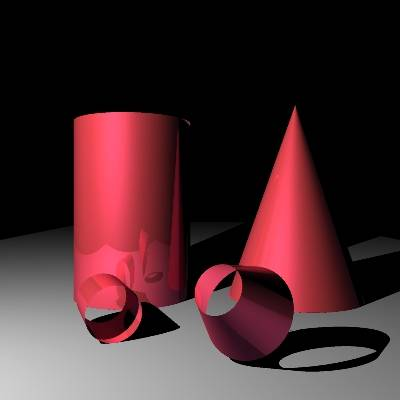# What Is The Difference Between A Cylinder And A Cone?

A Cylinder and a Cone are different three dimensional shapes. The common factor between them is that they both have a circle shape as one of their dimensions, on one side for a Cone, and two sides on a Cylinder. The sides of a Cylinder and Cone are curved, not flat. A Cylinder has two circle bases while a Cone only has one. See below for a picture showing the visual differences.An example of an every day life example of a Cone shape form is in coffee machine funnels or ice cream cones. An example of an every day life example of a Cylinder shape form is a glass or can of baked beans or soup.

The formula for finding the area of a Cone is also different from that of finding the area of a Cylinder.

The formula for finding the area of a Cone = curved surface = πrL + base = πr2

The formula for finding the area of a Cylinder = (area of bases) 2πr2 + 2πrh (area of side)

The formula for finding the volume of a Cone is also different from that of finding the area of a Cylinder.

The formula for finding the volume of a Cone = 1/3πr2h

The formula for finding the volume of a Cylinder = πr2h

thanked the writer.